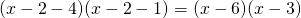# Solution assignment 02 The basics

### Assignment 2

Factorize: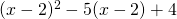### Solution

We can work out this formula resulting in a standard quadratic function and next try to to factorize the result. In this case it is faster to use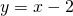. Then we get the following equation: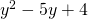This can be factorized as follows: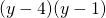which can be rewritten: